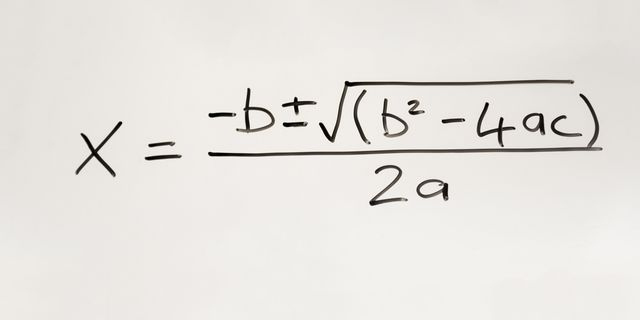06 Feb 2020

# Getting stuck on quadratic equations? Consider using this trick.Using a calculator program is still the best way to solve questions involving quadratics on the SAT, ACT, and SAT Subject Tests in Math levels 1 and 2 (here’s a video showing how you can do this on a few different standard-issue graphing calculators).

However, if you aren’t using a graphing calculator, or if programming one isn’t an option, this technique is a great way to solve quadratics.

Solving quadratic equations using the quadratic formula is often time consuming, and the long formula can be difficult for students to memorize. Or, students are taught to factor out the expression and use trial and error to solve. This strategy can end up being an inefficient use of your time during a timed exam.

It turns out there is a better way–and, it even works for equations that are not easily factorable.

In this article from the New York Times, A Carnegie Mellon Professor of Mathematics describes a new, surprisingly intuitive method to solve quadratic equations.

Written and video tutorials of the process can also be found directly from Dr. Loh’s blog, through this link.

Happy solving!